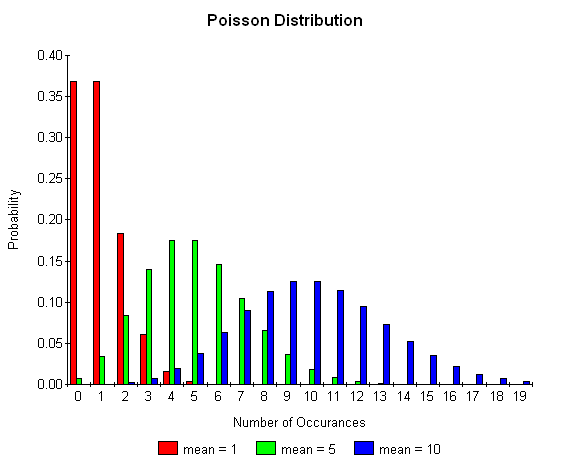``````#include <boost/math/distributions/poisson.hpp>
namespace boost { namespace math {

template <class RealType = double,
class Policy   = policies::policy<> >
class poisson_distribution;

typedef poisson_distribution<> poisson;

template <class RealType, class Policy>
class poisson_distribution
{
public:
typedef RealType value_type;
typedef Policy   policy_type;

poisson_distribution(RealType mean = 1); // Constructor.
RealType mean()const; // Accessor.
}

}} // namespaces boost::math``````

The Poisson distribution is a well-known statistical discrete distribution. It expresses the probability of a number of events (or failures, arrivals, occurrences ...) occurring in a fixed period of time, provided these events occur with a known mean rate λ (events/time), and are independent of the time since the last event.

The distribution was discovered by Simé on-Denis Poisson (1781 to 1840).

It has the Probability Mass Function:for k events, with an expected number of events λ.

The following graph illustrates how the PDF varies with the parameter λ:CautionThe Poisson distribution is a discrete distribution: internally functions like the `cdf`and `pdf` are treated "as if" they are continuous functions, but in reality the results returned from these functions only have meaning if an integer value is provided for the random variate argument.   The quantile function will by default return an integer result that has been rounded outwards. That is to say lower quantiles (where the probability is less than 0.5) are rounded downward, and upper quantiles (where the probability is greater than 0.5) are rounded upwards. This behaviour ensures that if an X% quantile is requested, then at least the requested coverage will be present in the central region, and no more than the requested coverage will be present in the tails.   This behaviour can be changed so that the quantile functions are rounded differently, or even return a real-valued result using Policies. It is strongly recommended that you read the tutorial Understanding Quantiles of Discrete Distributions before using the quantile function on the Poisson distribution. Thereference docs describe how to change the rounding policy for these distributions.

##### Member Functions

```poisson_distribution(RealType mean = 1);
```

Constructs a poisson distribution with mean mean.

```RealType mean()const;
```

Returns the mean of this distribution.

##### Non-member Accessors

All the usual non-member accessor functions that are generic to all distributions are supported: Cumulative Distribution FunctionProbability Density FunctionQuantile,Hazard FunctionCumulative Hazard Functionmeanmedianmodevariance,standard deviationskewnesskurtosiskurtosis_excessrange and support.

The domain of the random variable is [0, ∞].

##### Accuracy

The Poisson distribution is implemented in terms of the incomplete gamma functions gamma_p and gamma_q and as such should have low error rates: but refer to the documentation of those functions for more information. The quantile and its complement use the inverse gamma functions and are therefore probably slightly less accurate: this is because the inverse gamma functions are implemented using an iterative method with a lower tolerance to avoid excessive computation.

##### Implementation

In the following table λ is the mean of the distribution, k is the random variable, p is the probability and q = 1-p.

Function

Implementation Notes

pdf

Using the relation: pdf = e λk / k!

cdf

Using the relation: p = Γ(k+1, λ) / k! = gamma_q(k+1, λ)

cdf complement

Using the relation: q = gamma_p(k+1, λ)

quantile

Using the relation: k = gamma_q_inva(λ, p) - 1

quantile from the complement

Using the relation: k = gamma_p_inva(λ, q) - 1

mean

λ

mode

floor (λ) or ⌊λ⌋

skewness

1/√λ

kurtosis

3 + 1/λ

kurtosis excess

1/λ